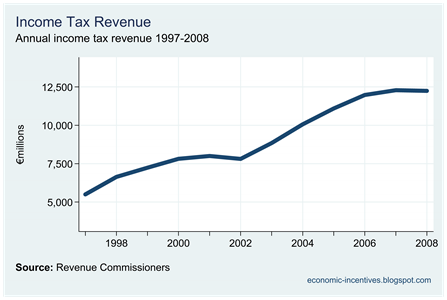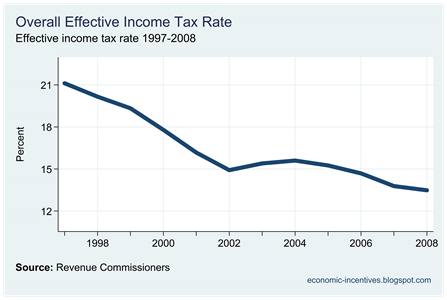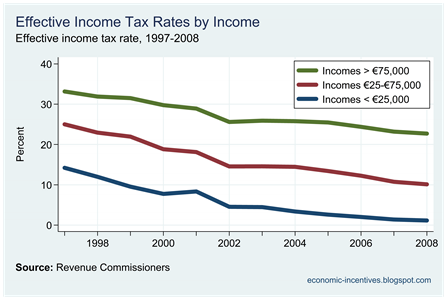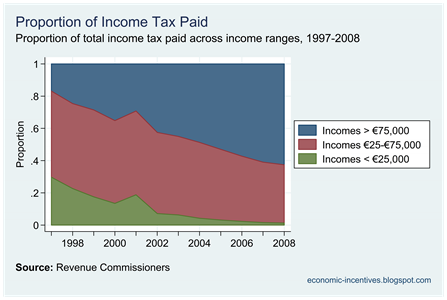## Sunday, March 13, 2011

### Effective Income Tax Rates

Since 1997 Income Tax Revenue rose on an almost annual basis from €5.5 billion in 1997 to €12.3 billion in 2008.At the same time, however, the changes introduced in the intervening budgets saw the actual effective rate of income tax fall.Using data from the Revenue Commissioners Annual Statistical Report shows that the effective rate of income tax applied to total gross income fell from 21.1% in 1997 to 13.5% in 2008.  These figures are derived from Table IDS1 from the Revenue’s Income Distribution Statistics and give the actual amount of tax collected as percentage of gross income.  If the 21% was applied to the 2008 gross income, tax revenue would have been €7 billion higher.

The statistics also allow us to examiner the effective rate on incomes in different ranges.  Here we will consider just three.

• Incomes under €25,000
• Incomes between €25,000 and €75,000
• Incomes over €75,000

The following graph gives the average effective rate applied to all gross incomes in these ranges.  It should be noted that these are not inflation-adjusted.  An income of IR£20,000 in 1998 (roughly equivalent to €25,000) would not be the same thing as an income of €25,000 in 2008 but we can use this to give a general guide to what has happened to effective tax rates to “low”, “middle” and “high” incomes.For all categories the effective rate has fallen.  In percentage points, the biggest fall was seen in the middle category which fell by 14.9 percentage points from 25.0% to 10.1%.  The effective rate on low incomes fell by 13.1 percentage points from 14.2% to 1.1% – unless it was to turn negative it could not really have fallen by much more.  The smallest percentage point drop was seen for high incomes which fell by 10.5 percentage points from 33.2% to 22.7%.

In proportionate terms the biggest fall occurred for low incomes with the effective income tax now over 90% lower than it was in 1997.  For middle incomes the effective income tax rate is now 60% lower than it was in 1997.  The smallest proportionate fall was for high incomes where the effective rate is 32% lower than it was in 1997.

Here is the proportion of the total income tax paid by each income group.This is not a true measure of the changing distribution of the income tax burden as part of the change is due to the change in incomes that has occurred since 1997.  A graph of the proportion of income earned by the three ranges used can be seen here.  A truer measure of the burden would to represent income deciles in the graph.  Maybe later.  The jump in 2001 is because this was a “short” tax year as the tax year was altered to comply with the calendar year.

In 1997 incomes under €25,000 accounted for 44.1% of aggregate gross income and paid 29.7% of the total income tax.  By 2008, such incomes accounted for 14.6% of income and paid 1.2% of the total income tax.

Income between €25,000 and €75,000 made up 45.1% of the aggregate income and paid 53.4% of the total tax.  The proportion of incomes from this range was 48.2% in 2008 but the proportion of tax paid had fallen to 36.2%.

Earnings for the high income bracket accounted for 10.8% of total income in 1997 and they paid  16.9% of the total tax.  By 2008 these earnings accounted for 37.2% of the total but they now paid 62.6% of the tax.

#### 1 comment:

1.At the moment People seem to be lashing out and complaining about various issues.
One Issue I seem to hearing regularly is that the socialist idae that the rich should be taxed more.
This article and its figures prove that the rich are paying more tax now than in the past. Not just more, but they have taken on a burden greater than their proportionate income.

My Question is a question based on projection and I understand that a definate answer cannot be given but maybe you would know a refferance article that tackles my question.

My question is this. If the Rich where taxed 75%. My guess is that they would take tax exile elsewhere or at least some would. How would their emmigration affect Irish entiprise? Would business be lost to other countries and how much so?

A Past Pupil.